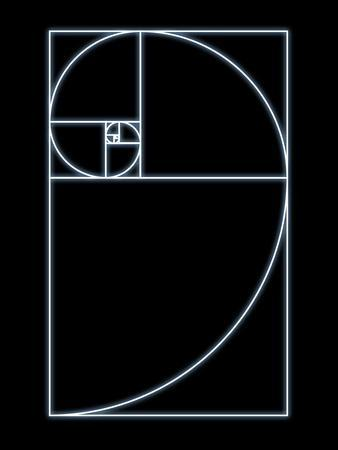Photographic Print

Fibonacci spiral Computer artwork of a spiral within squares whose sides decrease in length by a factor of This number phi is derived from the golden ratio Phi The spiral is formed by drawing a quarter circle in each box It resembles that seen in nature such as the shape of a snails shell or the segmentation of a pine cone The Fibonacci spiral was named after the Italian mathematician Leonardo Fibonacci and is also known as an equiangular or logarithmic spiral